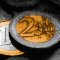Savings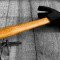DIY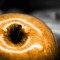Health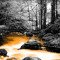EcologyScience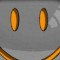Fun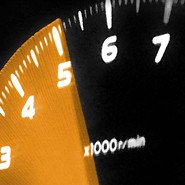# Calculate an average acceleration

Calculate the average acceleration of a object with a speed of departure and arrival.

m/s
m/s
seconds

m/s2

## Info

In physics, acceleration is the rate of change of velocity of an object with respect to time. An object's acceleration is the net result of all forces acting on the object, as described by Newton's Second Law. The SI unit for acceleration is metre per second squared (m s−2). Accelerations are vector quantities (they have magnitude and direction) and add according to the parallelogram law. The vector of the net force acting on a body has the same direction as the vector of the body's acceleration, and its magnitude is proportional to the magnitude of the acceleration, with the object's mass (a scalar quantity) as proportionality constant.
Source: Wikipedia